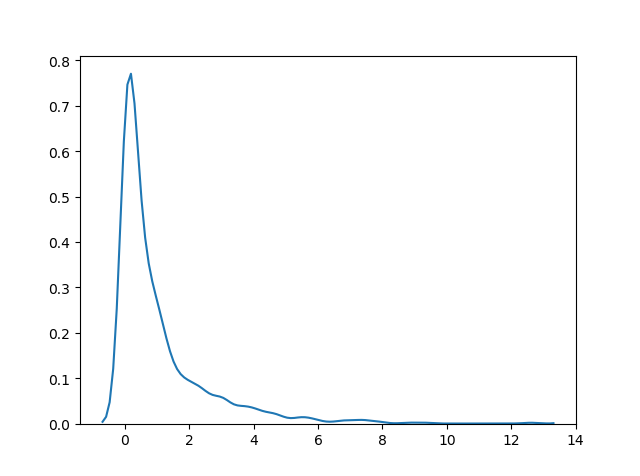THE WORLD'S LARGEST WEB DEVELOPER SITE

# Chi Square Distribution

## Chi Square Distribution

Chi Square distribution is used as a basis to verify the hypothesis.

It has two parameters:

`df` - (degree of freedom).

`size` - The shape of the returned array.

### Example

Draw out a sample for chi squared distribution with degree of freedom 2 with size 2x3:

from numpy import random

x = random.chisquare(df=2, size=(2, 3))

print(x)
Try it Yourself »

## Visualization of Chi Square Distribution

### Example

from numpy import random
import matplotlib.pyplot as plt
import seaborn as sns

sns.distplot(random.chisquare(df=1, size=1000), hist=False)

plt.show()

### ResultTry it Yourself »